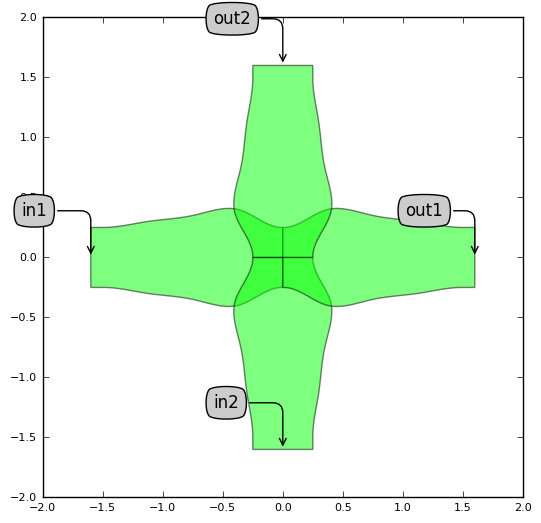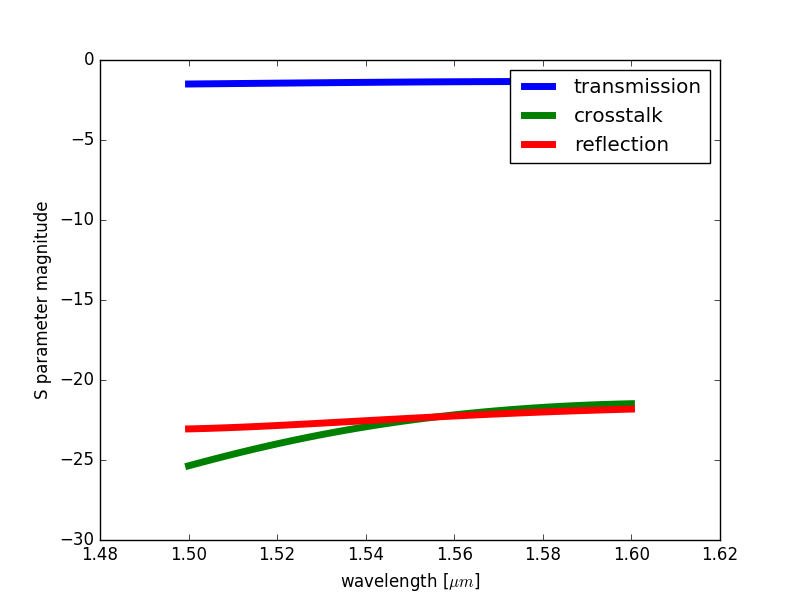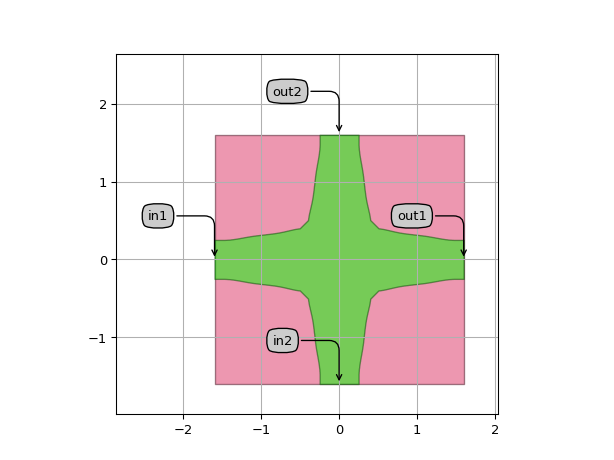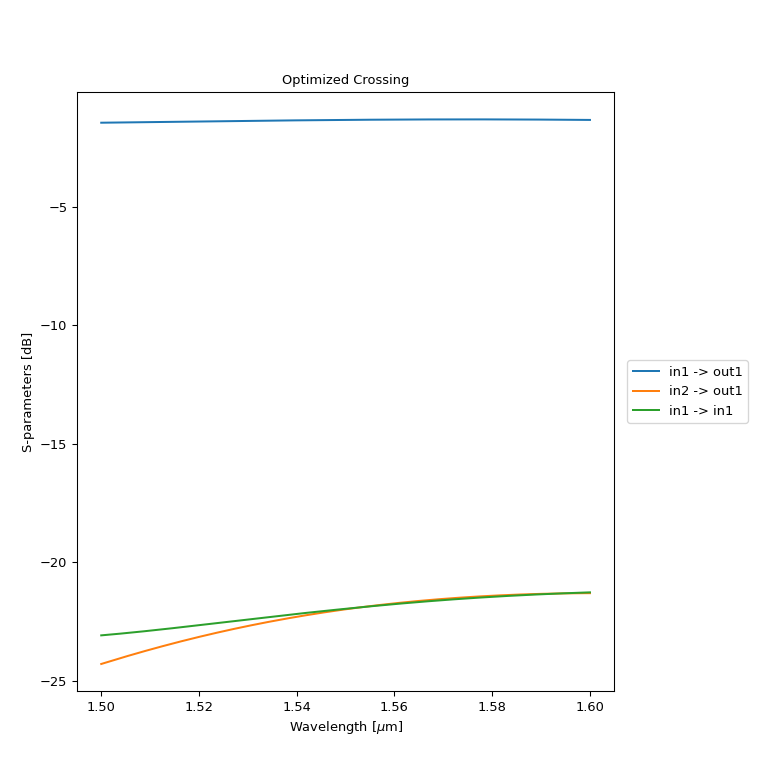# Waveguide crossing¶

SiFab contains a parametric crossing (Crossing) and an optimized crossing with fixed properties (CrossingOptimized) that has been simulated using a Lumerical simulation recipe.

## Crossing¶

This crossing is completely parametric and builds up from splines with equidistant waypoints, separated separated by segment_length. The height of the way-points is determined by widths. The crossing is calculated to be completely symmetric along the X and the Y axis. The parametric crossing has no circuit model.

from si_fab import all as pdk

# Parametric Crossing

crossing = pdk.Crossing(name="Crossing",
widths=[0.5, 0.8, 0.7, 0.8, 0.5],
segment_length=0.375,
min_straight=0.1,
discretisation=0.01)

crossing_lv = crossing.Layout()
crossing_lv.visualize(annotate=True)### Simulating a Crossing¶

Standalone simulations of the crossing can be done by applying the simulation recipe of the crossing onto a parametric layout. Most often this is done in a context of exploration or optimization. As simulations can take time to complete, it is best to use a structure where you create one folder where you save all your results for later inspections. This way you can run longer scripts once and process the data later.

si_fab/components/crossing/doc/example_crossing_simulation.py
import si_fab.all as pdk
from si_fab.components.crossing.simulation.simulate_lumerical import simulate_crossing
import joblib
import os
import pylab as plt
import numpy as np

inspect = False  # Inspect the simulation
resimulate = True  # Resimulate
project_folder = "./crossing_sim"  # Name of the project
smatrix_path = os.path.join(project_folder, "smatrix.z")  # Path where the smatrix is saved

if not os.path.exists(project_folder):
os.mkdir(project_folder)

cell = pdk.Crossing(name="Crossing")
lv = cell.Layout()
fig = lv.visualize(annotate=True, show=False)
fig.savefig(os.path.join(project_folder, "crossing_sim_layout.png"),
transparent=True,
bbox_inches='tight')

if resimulate:
smatrix = simulate_crossing(layout=lv, project_folder=project_folder, inspect=inspect)
joblib.dump(smatrix, smatrix_path)

if inspect is False:
wavelengths = smatrix.sweep_parameter_values

def dB(x): return 20 * np.log10(np.abs(x))
plt.figure()
plt.plot(wavelengths, dB(smatrix["out1", "in1"]), label='transmission', linewidth=5)
plt.plot(wavelengths, dB(smatrix["out1", "in2"]), label='crosstalk', linewidth=5)
plt.plot(wavelengths, dB(smatrix["in1", "in1"]), label='reflection', linewidth=5)
plt.legend()
plt.xlabel(r'wavelength [$\mu m$]')
plt.ylabel('S parameter magnitude')
plt.savefig(os.path.join(project_folder, "model.png"), transparent=True)## CrossingOptimized¶

CrossingOptimized is a pre-simulated component with locked properties. The simulated s-matrix is used by the circuit model to run Caphe simulations.

from si_fab import all as pdk
import pylab as plt
import numpy as np

# Optimized Crossing

crossing = pdk.CrossingOptimized()

crossing_lv = crossing.Layout()
crossing_lv.write_gdsii("crossing.gds")
crossing_lv.visualize(annotate=True)
crossing_cm = crossing.CircuitModel()

def dB(x): return 20 * np.log10(np.abs(x))

wavelengths_sim = np.linspace(1.50, 1.6, 1000)
smat = crossing_cm.get_smatrix(wavelengths=wavelengths_sim)
plt.figure()
plt.plot(wavelengths_sim, dB(smat["out1", "in1"]), label='transmission', linewidth=5)
plt.plot(wavelengths_sim, dB(smat["out1", "in2"]), label='crosstalk', linewidth=5)
plt.plot(wavelengths_sim, dB(smat["in1", "in1"]), label='reflection', linewidth=5)
plt.legend()
plt.title("Optimized Crossing")
plt.xlabel(r'wavelength [$\mu m$]')
plt.ylabel('S parameter magnitude')
plt.show()